# WPF Sudoku Grand Prix (Indian Round) : 9th - 11th February 2013Classic Sudoku   Place a digit from 1 to 9 in each empty cell so that each digit appears exactly once in each row, column and outlined region.1 Classic Sudoku   Place a digit from 1 to 9 in each empty cell so that each digit appears exactly once in each row, column and outlined region.2 Sudoku XV   Apply standard Sudoku rules.   Adjacent cells with two digits summing to 5 are marked by V, while those summing to 10 are marked by X. All possible X and V are marked.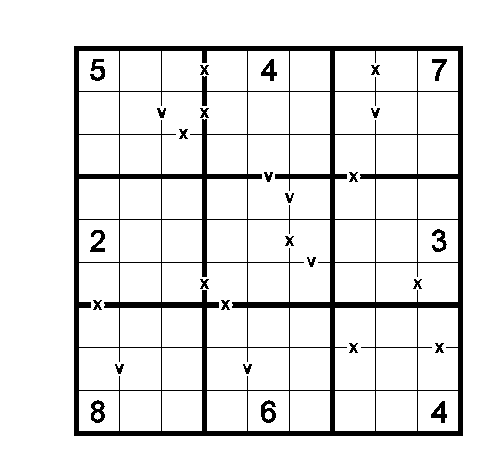3 Diagonal Sudoku   Apply standard Sudoku rules.   Additionally, digits do not repeat across main (marked) diagonals.4 Toroidal Sudoku   Apply standard Sudoku rules.   Some of the outlined regions wrap around the top and bottom edges, and/or the left and right edges of the grid.   (The example uses 1 to 7)5 Killer Sudoku   Apply standard Sudoku rules.   The sum of digits in cells inside every cage must equal the total given for the cage at the upper left cell. Each digit in the cage must be unique.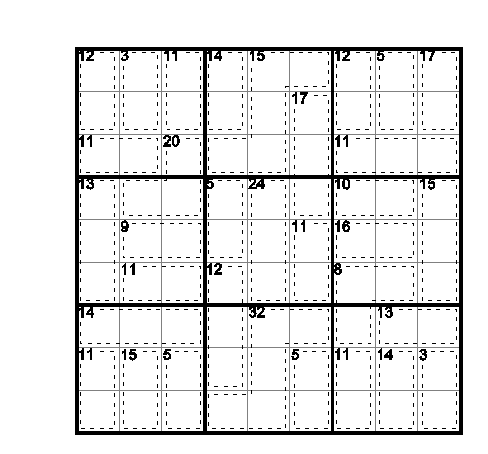6 Odd Sudoku   Apply standard Sudoku rules.   Additionally, shaded cells must contain odd (1 3 5 7 9) digits.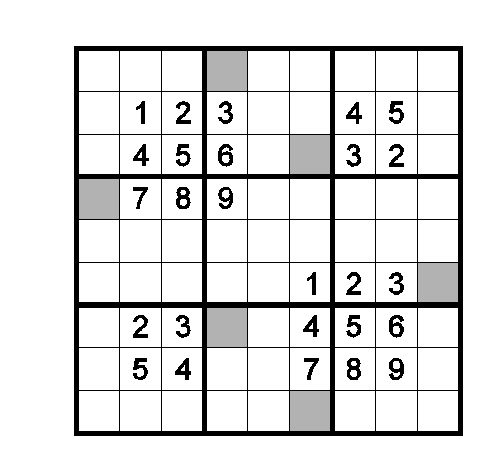7 Renban Groups   Apply standard Sudoku rules.   Additionally, shaded cells in each 3X3 box must contain consecutive digits.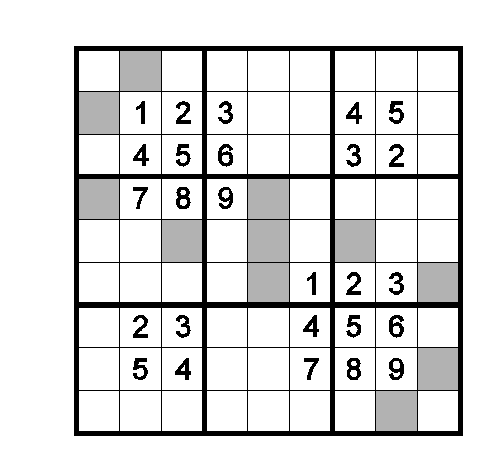8 Coded Pair   Apply standard Sudoku rules.   Some letters are given between two adjacent cells. Same letter represents same pair of digits across the grid. Different letters must represent different pairs. Ordering of digits inside the pair does not matter.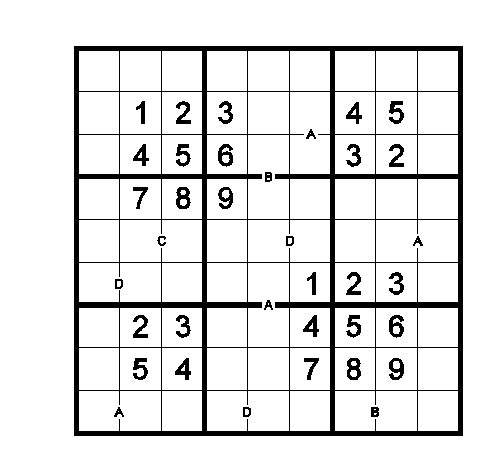9 Quad Sum Sudoku   Apply standard Sudoku rules.   A circle at a corner implies that one digit is sum of remaining 3 digits at that corner.10 Repeated Neighbours   Apply standard Sudoku rules.   Each shaded cell must have repeating orthogonal neighbours (neibhbours sharing an edge). White cells must have distinct orthogonal neighbours.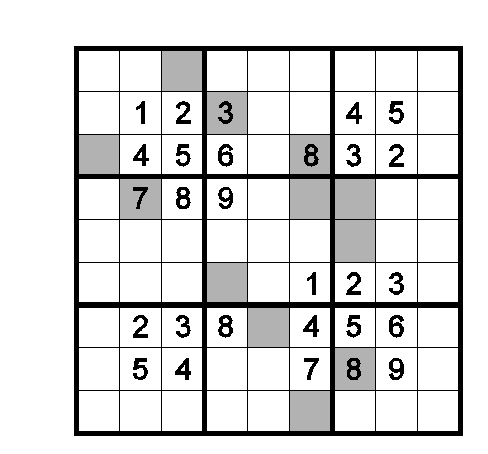11 Braille Sudoku   Apply standard Sudoku rules, except that 6 of 1-9 will be used in the grid. It is part of solving to figure out the 6 digits.   The Braille digits for 1 to 9 are given. Form the digits in Braille by adding dots to the diagram as needed. An extra work grid is provided.12 Thermo Sudoku   Apply standard Sudoku rules.   The digits in each “thermometer” shaped region must be strictly increasing from the circular “bulb” to the other end(s).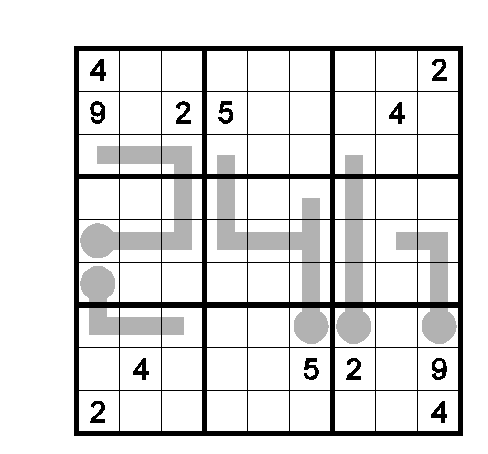13 Odd Even Big Small   Apply standard Sudoku rules.   Four different symbols outside the grid indicate four different properties of the first two cells in the along that row or column. The properties are  odd (1 3 5 7)  even (2 4 6 8)  big (5 6 7 8)  small (1 2 3 4).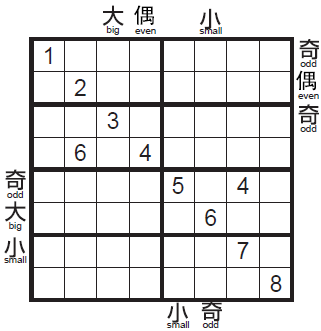15 Disjoint Groups   Apply standard Sudoku rules.   Additionally, no digit can appear in the same position in different 3X3 boxes.16 Odd Even Frame   Apply standard Sudoku rules.   Clue outside is either the sum of all odd digits in the first 3 cells or sum of all even digits in the first 3 cells.17 Point To Next   Apply standard Sudoku rules.   If digit 'n' is placed in a cell with arrow, digit 'n+1' must be placed in one of the cells pointed by the arrow.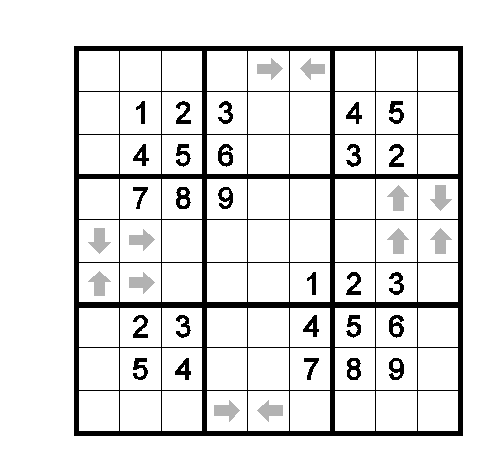19 Linked Classics   Apply standard Sudoku rules.   The two grids are linked: every row has an exact duplicate in the other grid.   (The example uses 6X6 grid.)20